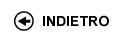Politecnico di Torino
04KXVLI, 04KXVJM, 04KXVLJ, 04KXVLL, 04KXVLM, 04KXVLN, 04KXVLS, 04KXVLX, 04KXVLZ, 04KXVMA, 04KXVMB, 04KXVMC, 04KXVMH, 04KXVMK, 04KXVMN, 04KXVMO, 04KXVMQ, 04KXVNX, 04KXVNZ, 04KXVOA, 04KXVOD, 04KXVPC, 04KXVPI, 04KXVPL
Physics I
Corso di Laurea in Ingegneria Dell'Autoveicolo (Automotive Engineering) - Torino
Corso di Laurea in Ingegneria Meccanica (Mechanical Engineering) - Torino
Corso di Laurea in Ingegneria Delle Telecomunicazioni (Telecommunications Engineering) - Torino
Espandi...
 Docente Qualifica Settore Lez Es Lab Tut Anni incarico Iazzi Felice76 18 6 0 14
 SSD CFU Attivita' formative Ambiti disciplinari FIS/01 10 A - Di base Fisica e chimica
Esclusioni:
17AXO
 Programma INTRODUCTION: physical quantities and units of measurement The measurement process. Units of measurement and dimensions of physical observables. Particle KINEMATICS. Review of vector calculus. Reference frames. Position, displacement, velocity, and acceleration in 1, 2 and 3 dimensions. Uniform motion. Motion with constant and variable acceleration. Polar and cylindrical coordinates. Tangent and normal components of acceleration, radius of curvature. Circular motion. Velocity and acceleration composition laws. Particle DYNAMICS: Newton�s Laws, Work and Energy. Mass and force. Inertial reference frames. The three Newton laws. Gravitational force. Coulomb�s force. Elastic force. Constraints. Static and kinetic friction. Viscous resistance. Non inertial reference frames: fictitious forces. Work and kinetic energy: definition of work, work-energy theorem. Potential Energy and energy conservation: conservative force fields and potential energy. Mechanical-energy conservation. Examples and applications. Harmonic oscillator: harmonic motion, damped and driven harmonic motion. Resonance. Linear momentum and angular momentum: impulse-momentum theorem. Moment of a force (torque) and angular momentum. Angular momentum theorem. Newton�s Law of Gravitation and Coulomb�s Law. Law of universal gravitation, inertial and gravitational mass. Coulomb�s law and charge. Superposition principle of forces. Gravitational and electrostatic fields. Field lines and flux. Gravitational and electrostatic potential: Gauss� theorem, charge distributions with spherical symmetry and other examples. Kepler�s laws. DYNAMICS and STATICS of many-particle systems and COLLISIONS. Continuous and discrete systems. Internal and external forces. Equation of motion of the center of mass. Total momentum of many-particle systems. Center of mass and linear momentum conservation. Systems with variable mass. Angular momentum of many-body systems: Angular momentum theorem and conservation. Angular momentum and kinetic energy in the center-of-mass frame. Collisions: momentum and kinetic energy in collision processes. Elastic and inelastic collisions. DYNAMICS of a rigid body. Definition of rigid body. Traslation and rotation about a fixed axis of a rigid body. Moment of inertia. Parallel-axis theorem. Rigid-body kinetic energy. Pure rolling motion. Rolling motion with slipping. Conservation laws in the rigid-body motion. Mechanical equilibrium of a rigid body. Examples and applications. FLUIDS. Pressure. Statics of fluids: hydrostatic pressure (Stevin�s law). Pascal�s law and Archimedes principle. Dynamics of ideal fluids: streamlines and streamtube. Equation of continuity. Bernoulli�s theorem. Examples and applications. Viscous fluids. THERMODYNAMICS: calorimetry, First Law of Thermodynamics and ideal gases. Basic concepts in calorimetry and thermometry. Heat transfer. Thermodynamic equilibrium and state variables. Reversible and irreversible thermodynamic transformations. Adiabatic, isothermal, isobaric and isochoric transformations. Ideal (or perfect) gases. Kinetic theory of gases, work and internal energy. First Law of Thermodynamics. Applications of the first law to ideal gases. THERMODYNAMICS: Second Law of Thermodynamics and Entropy. Second Law of Thermodynamics: Kelvin and Clausius statements. Heat engines and refrigerators. Thermal efficiency. Carnot�s cycle and other cycles. Carnot�s theorem. Thermodynamic temperature. Clausius� theorem. Entropy. Criteri, regole e procedure per l'esame The goal of the exam is to test the knowledge of the candidate about the topics included in the official program of Physics 1 and to verify the skill in solving problems. The exam consists of two steps: a written exam followed by an obligatory oral exam. The assessment of both the written and the oral part is based on marks ranging from 0 to 30 (the maximum is 30 out of 30 cum laude). The final assessment is determined by the average of the marks obtained in the written exam and the interview. Written exam: a mark less than 16 out of 30 in the written exam is not sufficient for the admission to the oral exam. The exam actually begins when a candidate enters the exam room. The exam of a candidate is recorded with "rejected" in two cases: If a candidate decides to stop his written exam once the exam has started and if the assessment of his written exam is less than 16 marks out of 30. Candidates are not allowed to take in the exam room text-books or notes relevant to the Physics-1 program. The use of electronic calculators is allowed provided these are cleared of all pre-stored programmes or information. The written exam consists of 3-4 questions. In general, these are exercises with the same degree of difficulty of the exercises discussed in the Physics-1 lectures devoted to applications (esercitazioni). Part of these questions, however, might be focused on the theory included in the Physics-1 program. The exercises proposed in this exam are inspired by the exercises/examples contained in the textbook used by the course lecturer. The text-book will be indicated by the lecturer at the beginning of the course. Oral exam: students are admitted to the oral exam if the assessment of their written exam is 16 marks out of 30 or more. The assessment of oral exam cannot be less than 18 marks out of 30. The exam is passed if the final assessment (average of the marks of the written and oral exam) is 18 marks out of 30 or more. Oral exam is mainly oriented to check whether a candidate has a sufficiently wide knowledge of the theory of the Physics-1 program. Oral exam may include questions concerning the written exam of the candidate and his activity in the physics laboratory. The theoretical topics discussed in the course lectures are summarized in the program of Physics-1 courses of the Politecnico. In general, the oral exam must be passed in the same exam session (appello) in which the written exam is passed. Orario delle lezioni Statistiche superamento esami Programma definitivo per l'A.A.2010/11